# 1097 Results

View
Selected filters:
• Common Core State Standards MathOnly Sharing Permitted
CC BY-NC-ND
Rating

This lesson unit is intended to help teachers assess how well students are able to visualize two-dimensional cross-sections of representations of three-dimensional objects. In particular, the lesson will help you identify and help students who have difficulties recognizing and drawing two-dimensional cross-sections at different points along a plane of a representation of a three-dimensional object.

Subject:
Geometry
Material Type:
Assessment
Lesson Plan
Provider:
Shell Center for Mathematical Education
U.C. Berkeley
Provider Set:
Mathematics Assessment Project (MAP)
04/26/2013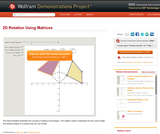Conditional Remix & Share Permitted
CC BY-NC-SA
Rating

This Demonstration illustrates the concept of rotating a 2D polygon. The rotation matrix is displayed for the current angle. The default polygon is a square that you can modify.

Subject:
Geometry
Material Type:
Activity/Lab
Diagram/Illustration
Homework/Assignment
Interactive
Simulation
Teaching/Learning Strategy
Provider:
Wolfram Research
Provider Set:
Wolfram Demonstrations Project
Author:
Mito Are and Valeria Antohe
09/04/2013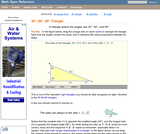Rating

An interactive applet and associated web page that demonstrate the properties of a 30-60-90 triangle. The applet shows a right triangle that can be resized by dragging any vertex. As it is dragged, the remaining vertices change so that the triangle's angles remain 30 degrees, 60 degrees and 90 degrees The text on the page points out that the sides of a 30-60-90 triangle are always in the ratio of 1 : 2 : root 3 Applet can be enlarged to full screen size for use with a classroom projector. This resource is a component of the Math Open Reference Interactive Geometry textbook project at http://www.mathopenref.com.

Subject:
Geometry
Material Type:
Simulation
Provider:
Math Open Reference
Author:
John Page
02/16/2011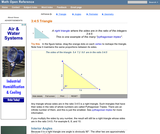Rating

An interactive applet and associated web page that demonstrate the properties of a 3:4:5 triangle - one of the Pythagorean triples. The applet shows a right triangle that can be resized by dragging any vertex. As it is dragged, the remaining vertices change so that the triangle's side remain in the ration 3:4:5. The text on the page has an example of how the triangle can be used to measure a right angle on even large objects. Applet can be enlarged to full screen size for use with a classroom projector. This resource is a component of the Math Open Reference Interactive Geometry textbook project at http://www.mathopenref.com.

Subject:
Geometry
Material Type:
Simulation
Provider:
Math Open Reference
Author:
John Page
02/16/2011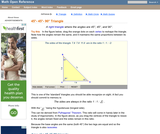Rating

An interactive applet and associated web page that demonstrate the properties of a 45-45-90 isosceles right triangle. The applet shows a right triangle that can be resized by dragging any vertex. As it is dragged, the remaining vertices change so that the triangle's angles remain 45 degrees, 45 degrees and 90 degrees The text on the page points out that the sides of a 45-45-90 triangle are always in the ratio of 1 : 2 : root 2 Applet can be enlarged to full screen size for use with a classroom projector. This resource is a component of the Math Open Reference Interactive Geometry textbook project at http://www.mathopenref.com.

Subject:
Geometry
Material Type:
Simulation
Provider:
Math Open Reference
Author:
John Page
02/16/2011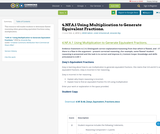Conditional Remix & Share Permitted
CC BY-NC-SA
Rating

This resource will enable students to determine flawed reasoning when generating equivalent fractions using multiplication.

Subject:
Mathematics
Material Type:
Activity/Lab
Assessment
Homework/Assignment
Author:
Linda Schoenbrodt
Amanda Ray
10/04/2018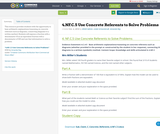Conditional Remix & Share Permitted
CC BY-NC-SA
Rating

This resource provides students with the opportunity to base arithmetic explanations/reasoning on concrete referents such as diagrams, connecting diagrams to a written method. Students will express a fraction with a denominator 10 as an equivalent fraction with a denominator of 100 and use that information to solve a problem.

Subject:
Mathematics
Material Type:
Activity/Lab
Assessment
Homework/Assignment
Author:
Linda Schoenbrodt
Amanda Ray
10/04/2018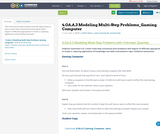Conditional Remix & Share Permitted
CC BY-NC-SA
Rating

This resource provides students with the opportunity to solve a multi-step contextual word problem with a degree of difficulty appropriate to Grade 4, requiring application of knowledge and skills.

Subject:
Mathematics
Material Type:
Activity/Lab
Assessment
Homework/Assignment
Author:
Linda Schoenbrodt
Amanda Ray
10/04/2018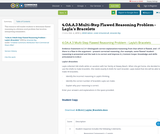Conditional Remix & Share Permitted
CC BY-NC-SA
Rating

This resource will enable students to determine flawed reasoning in a division word problem that involves interpreting remainders.

Subject:
Mathematics
Material Type:
Activity/Lab
Assessment
Homework/Assignment
Author:
Linda Schoenbrodt
Amanda Ray
10/04/2018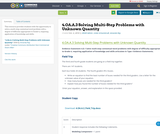Conditional Remix & Share Permitted
CC BY-NC-SA
Rating

This resource provides students with the opportunity to solve a multi-step contextual word problem with a degree of difficulty appropriate to Grade 4, requiring application of knowledge and skills.

Subject:
Mathematics
Material Type:
Activity/Lab
Assessment
Homework/Assignment
Author:
Linda Schoenbrodt
Amanda Ray
10/04/2018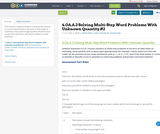Conditional Remix & Share Permitted
CC BY-NC-SA
Rating

This resource requires students to present the solution to a multi-step problem in the form of valid chains of reasoning, using symbols appropriately. Students must use the four operations with whole numbers to solve problems.

Subject:
Mathematics
Material Type:
Activity/Lab
Assessment
Homework/Assignment
Author:
Linda Schoenbrodt
Amanda Ray
10/04/2018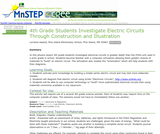Conditional Remix & Share Permitted
CC BY-NC-SA
Rating

As an activity related to FOSS unit Magnetism and Electricity, 4th grade science students use a computer download to explore electrical circuits and to generate illustrations of electrical circuits for physical models built in class

Subject:
Electronic Technology
Material Type:
Activity/Lab
Provider:
Science Education Resource Center (SERC) at Carleton College
Provider Set:
Pedagogy in Action
Author:
Lorraine Aaland
08/16/2012Conditional Remix & Share Permitted
CC BY-NC-SA
Rating

In this engineering, math, and sustainability project students answer the question, “Can I ride 53 miles on a bike from the energy of a single burrito?” They must define their variables, collect and analyze their data, and present their results. By the end of this project, developed by Allen Distinguished Educator Mike Wierusz, students should have all the information they need to design a burrito that would provide them with the exact caloric content necessary to ride 53 miles.

Subject:
Mathematics
Material Type:
Activity/Lab
Lesson Plan
Provider:
Allen Distinguished Educators
07/27/2016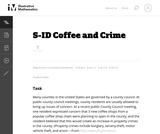Unrestricted Use
CC BY
Rating

This task addresses many standards regarding the description and analysis of bivariate quantitative data, including regression and correlation. Students should recognize that the pattern shown is one of a strong, positive, linear association, and thus a correlation coefficient value near +1 is plausible. Students should also be able to interpret the slope of the least-squares line as an estimated increase in y per unit change in x (and thus for a 3 unit increase in x, students should expect an estimated increase in y that equals 3 times the model's slope value).

Subject:
Mathematics
Material Type:
Activity/Lab
Provider:
Illustrative Mathematics
Provider Set:
Illustrative Mathematics
Author:
Illustrative Mathematics
02/19/2013Unrestricted Use
CC BY
Rating

This is a task from the Illustrative Mathematics website that is one part of a complete illustration of the standard to which it is aligned. Each task has at least one solution and some commentary that addresses important asects of the task and its potential use. Here are the first few lines of the commentary for this task: A seven-year-old boy has a favorite treat, Super Fruity Fruit Snax. These "Fruit Snax" come in pouches of 10 snack pieces per pouch, and the pouches ar...

Subject:
Mathematics
Material Type:
Activity/Lab
Provider:
Illustrative Mathematics
Provider Set:
Illustrative Mathematics
Author:
Illustrative Mathematics
05/22/2013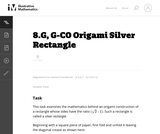Unrestricted Use
CC BY
Rating

This is a task from the Illustrative Mathematics website that is one part of a complete illustration of the standard to which it is aligned. Each task has at least one solution and some commentary that addresses important asects of the task and its potential use. Here are the first few lines of the commentary for this task: This task examines the mathematics behind an origami construction of a rectangle whose sides have the ratio $(\sqrt{2}:1)$. Such a rectangle is called ...

Subject:
Mathematics
Material Type:
Activity/Lab
Provider:
Illustrative Mathematics
Provider Set:
Illustrative Mathematics
Author:
Illustrative Mathematics
10/14/2013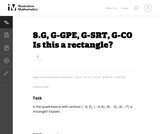Unrestricted Use
CC BY
Rating

This is a task from the Illustrative Mathematics website that is one part of a complete illustration of the standard to which it is aligned. Each task has at least one solution and some commentary that addresses important asects of the task and its potential use. Here are the first few lines of the commentary for this task: Is the quadrilateral with vertices $(-6, 2)$, $(-3,6)$, $(9, -3)$, $(6,-7)$ a rectangle? Explain....

Subject:
Mathematics
Material Type:
Activity/Lab
Provider:
Illustrative Mathematics
Provider Set:
Illustrative Mathematics
Author:
Illustrative Mathematics
03/13/2013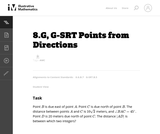Unrestricted Use
CC BY
Rating

This is a task from the Illustrative Mathematics website that is one part of a complete illustration of the standard to which it is aligned. Each task has at least one solution and some commentary that addresses important asects of the task and its potential use. Here are the first few lines of the commentary for this task: Point $B$ is due east of point $A$. Point $C$ is due north of point $B$. The distance between points $A$ and $C$ is $10\sqrt 2$ meters, and \$\angle BAC...

Subject:
Mathematics
Material Type:
Activity/Lab
Provider:
Illustrative Mathematics
Provider Set:
Illustrative Mathematics
Author:
Illustrative Mathematics
03/29/2013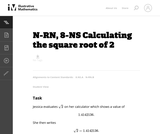Unrestricted Use
CC BY
Rating

This task is intended for instructional purposes so that students can become familiar and confident with using a calculator and understanding what it can and cannot do. This task gives an opportunity to work on the notion of place value (in parts (b) and (c)) and also to understand part of an argument for why the square root of two is not a rational number.

Subject:
Mathematics
Material Type:
Activity/Lab
Provider:
Illustrative Mathematics
Provider Set:
Illustrative Mathematics
Author:
Illustrative Mathematics